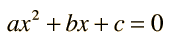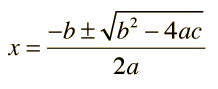The solution to a quadratic equation that has been placed in the standard formis given by the quadratic formulaEnter values for the coefficients and click outside the data boxes to initiate the calculation.

a = , b = , c =

The quantity b2 - 4ac =

For b2 ≥ 4ac there are two real roots:

x+ = and x- =

If b2 < 4ac then the roots are complex numbers:

x+ = + j

and its complex conjugate x- = - jPhysical applications of the quadratic formula Ballistic trajectories
Index

 HyperPhysics****HyperMath*****Algebra R Nave
Go Back